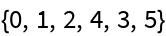#Function Repository Resource:

# ToInversionVector

Get the inversion vector of a permutation written as a list

Contributed by: Wolfram Staff
 ResourceFunction["ToInversionVector"][p] gives the inversion vector of the permutation list p.

## Details and Options

The inversion vector of a permutation of length n lists the number of times k is preceded by an entry greater than k, where k runs from 1 to n.

## Examples

### Basic Examples

This permutation has three numbers greater than 1 before 1, two numbers greater than 2 before 2 and so on:

 In:=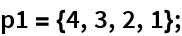Therefore, this is its inversion vector:

 In:=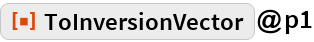Out=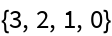Here is another example:

 In:=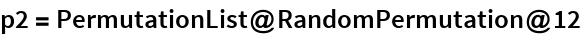Out=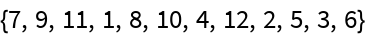In:=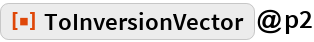Out=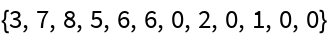### Scope

Here are all six permutation of {1,2,3}:

 In:=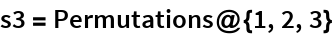Out=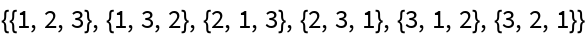Here are the corresponding inversion vectors:

 In:=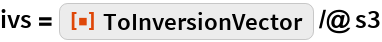Out=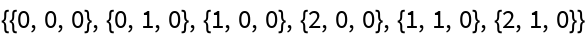In the factorial base, ivs represents the numbers from 0 to 3!-1:

 In:=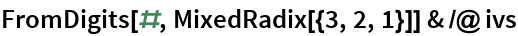Out=